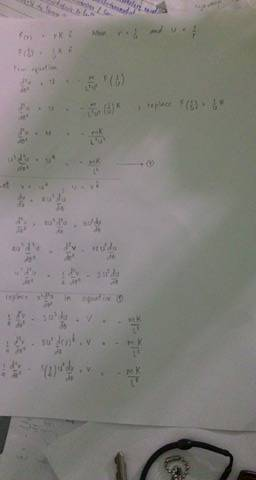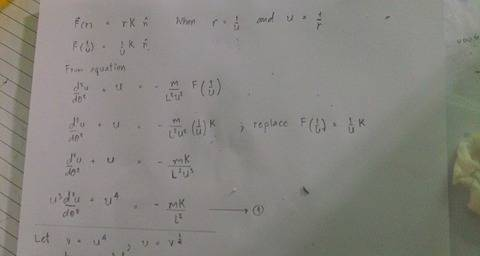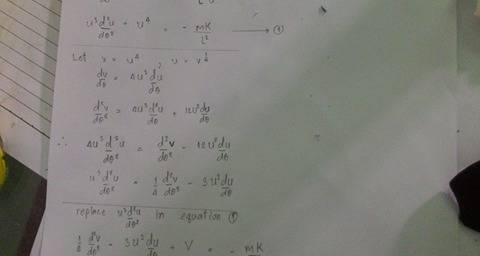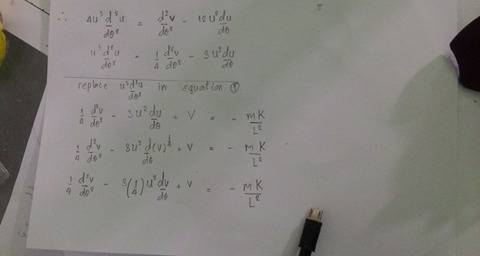# Problem ODE from mechanics equation

The particles are moving under force F = rK
when r = 1/u and u = 1/r

I tried to solve the problem by defining a variable v = u4 and u = v1/2

But I can not divide variable v out of variable u

I want to find r (Radius) of orbit#### Attachments

The particles are moving under force F = rK
when r = 1/u and u = 1/r

I tried to solve the problem by defining a variable v = u4 and u = v1/2

But I can not divide variable v out of variable u

I want to find r (Radius) of orbit

View attachment 213844#### Attachments

haruspex
Homework Helper
Gold Member
2020 Award
The particles are moving under force F = rK
when r = 1/u and u = 1/r
I cannot make sense of the question. What do r, K and u represent? Which way does the force act?
Please state the entire question exactly as given to you. If it is a translation, try to be as complete and accurate as you can.

The ability to post images is really for diagrams and textbook extracts. Please take the trouble to type in your equations. The first image you posted was unreadabe.